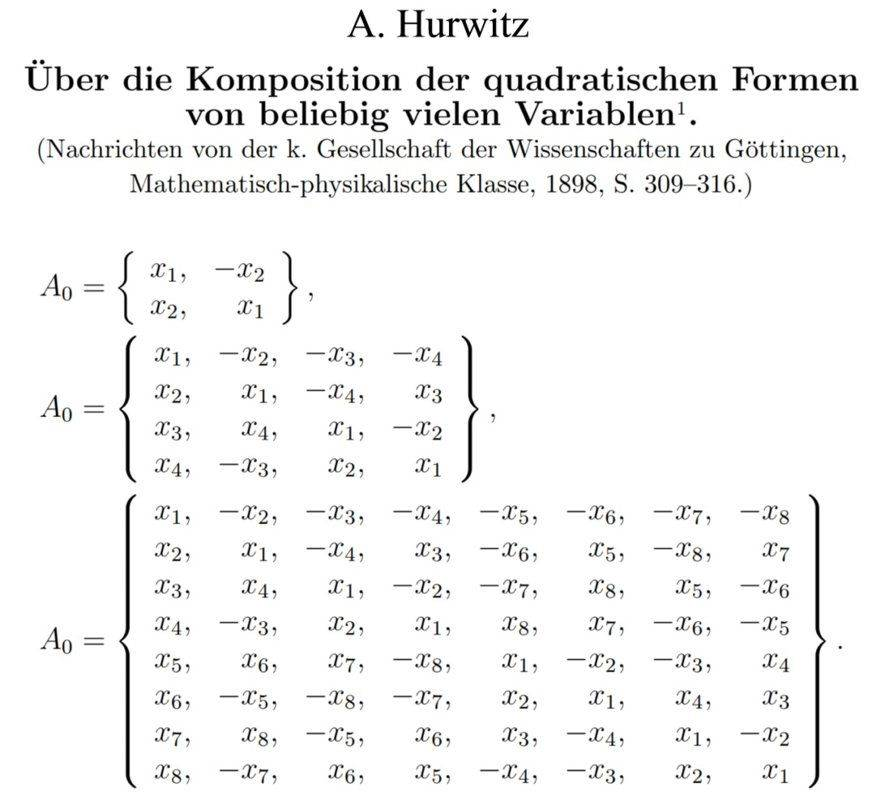# Atiyah's arithmetic physics

Gold Member
What is R C O H in this context?

Auto-Didact
What is R C O H in this context?
Real Complex Octonion Quaternion

•ohwilleke
lpetrich
Or in order of object size, R C H O.

These are the first four Cayley-Dickson algebras, starting with the real numbers and with each one of the others being produced from pairs of the elements of the previous one. The next one is he sedenions (size 16): S?

The algebras are rings with unity and with a conjugation operation that is a self-inverse (involution): ##(x^*)^* = x##. Each one is constructed from the previous one, if present, with operations
• Addition: ##(a,b) + (c,d) = (a+c, b+d)## (component-by-component)
• Multiplication: ##(a,b) (c,d) = (ac - d^*b, da + bc^*)##
• Conjugation: ##(a,b)^* = (a^*,-b)##
One can define a norm with ##xx^* = x^*x##. Applying to ##(a,b)## gives ##(aa^* + bb^*,0)##, and repeating gives ##xx^* = (\|x\|, 0, 0, \dots 0) = \|x\| (1, 0, 0, \dots 0)## where ##\|x\| = \sum_i (x_i)^2##. Every element with nonzero norm has a reciprocal: ##x^{-1} = x^* / \|x\|##.

The real numbers have a complete set of properties, and higher -ions gradually drop them. Their multiplication is commutative and associative, and interchangeable with norming, and they are self-conjugate.

Complex numbers lose self-conjugacy.

Quaternions lose multiplication commutativity.

Octonions lose multiplication associativity, though they have a form of partial associativity known as alternativity: ##(xx)y = x(xy)##, ##(xy)x = x(yx)##, and ##(yx)x = y(xx)##.

Sedenions lose multiplication alternativity, though they have a limited form of associativity known as power-associativity: ##x^m x^n = x^{m+n}##, where m and n can be negative as well as nonnegative integers. They also lose product-norm interchange. In general, ##\|x y\| \ne \|x\| \|y\|##. They also acquire nontrivial divisors of zero.

Higher -ions have no change in properties from sedenions.

•eloheim and ohwilleke
lpetrich
This has the consequence that quaternion multiplication can be represented by matrix multiplication, while octonion multiplication cannot.

The algebras' isomorphism groups are: R: identity group, C: Z2, H: SO(3), O and higher: G2. This seems related to the Standard Model with:
• U(1) - complex numbers
• SU(2) - quaternions
• SU(3) - subalgebra of G2, automorphisms of octonions
Is that the connection of this algebra sequence to the Standard Model?

•ohwilleke
Gold Member
What is R C O H in this context?

Hmm, RCHO, to preserve the order, sorry.

In the context of the interview with Atiyah, I am not sure if he wants to play with the proyective planes, which is a very usual bussiness in this field, or just with the straighforward ones. Relevant answer:

MA said:
Exactly so. The non-commutativity of the quaternions is at the heart of the problem I deal with in my calculation of α. The non-associativity of the octonions is much harder and will be in my next paper. Gravity is much harder than gauge theories of compact Lie groups. The division algebras and the physical forces are a perfect fit. Let me explain. The compact groups that act on ℝ2 , ℂ2 , ℍ2 are SO(2), U(2), U(3). The first gives electromagnetism, the second gives the electroweak theory and the subgroup SU(3) is the gauge group of strong interactions. But 𝕆2 is acted on by octonions which do not give a group because they are non-associative. That is why gravity is harder than gauge theories.

•ohwilleke and Auto-Didact
lpetrich
•Hans de Vries and Auto-Didact
Gold Member
Meaning algebras R2, C2, H2, and O2. R2 gives us SO(2) ~ U(1), C2 gives us U(2) ~ SU(2) * U(1), and H2 gives us SO*(4) ~ SU(2) * SL(2,R), not SU(3).

[URL='https://www.physicsforums.com/insights/author/john-baez/']John Baez on Octonions[/URL] mentions some ways of getting around octonions' non-associativity, IIRC.

This is from Hurwitz's original '##\mathbb{RCHO}##' paper:Note that these arrays can be read in two ways:

1) ... As a multiplication table of the generators.
2) ... As a 2x2, 4x4 or 8x8 matrix where each matrix represents a generator.

In case of 1) for example ##x_3## represents the (entire) generator and the table shows the product of the generator of the first row multiplied with the generator in the first column.

In case of 2) the matrix representing ##x_3## has a +1 for each position which contains ##x_3##, a -1 for each position which contains ##-x_3## and a zero for all other positions.

in case of ##\mathbb{C}## and ##\mathbb{H}## each generator is represented by its matrix. The matrices form a group. All matrices are square roots of -I and all matrices anti-commute with each other (except with the I matrix) and they are associative.

Not so with ##\mathbb{O}## however. Both the generators and matrices still anti-commute with each other but:

In case of 1) the generators form a group but are not associative.
In case of 2) the matrices are associative (as matrices are always associative) but they do not form a group anymore.

In the later case the 8x8 matrices can be used to set up the space of a 7-sphere like the 4x4 matrices can be used for a 3-sphere, so:

$$\big(ax_2+bx_3+cx_4+dx_5+ex_6+fx_7+gx_8\big)^2 = -\big(a^2+b^2+c^2+d^2+e^2+f^2+g^2\big)I$$

Last edited:
•Auto-Didact